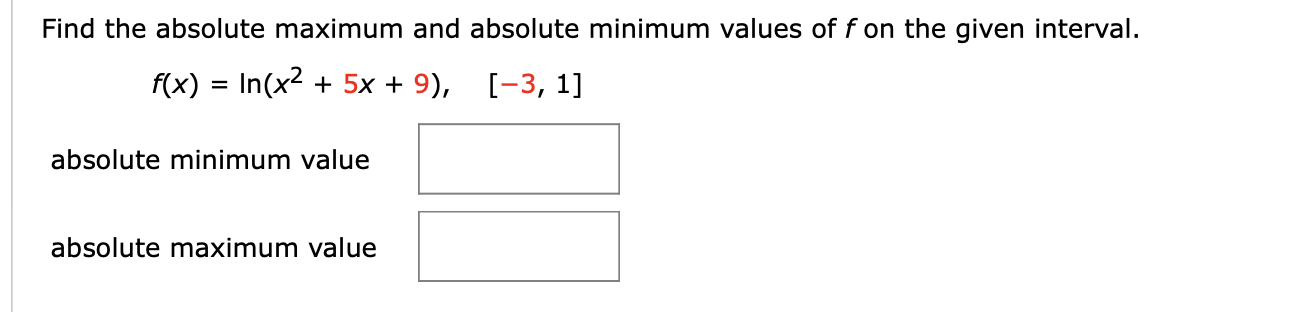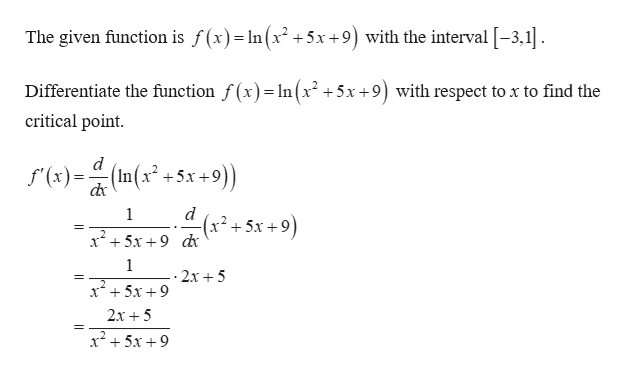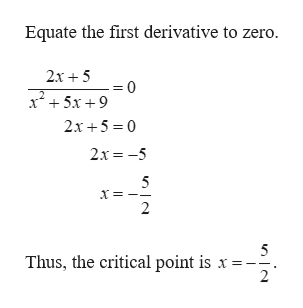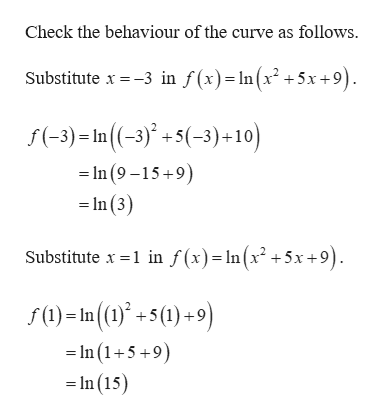# Find the absolute maximum and absolute minimum values of f on the given interval.f(x) In(x2 5x 9), [-3, 1]absolute minimum valueabsolute maximum value

Question
203 viewshelp_outlineImage TranscriptioncloseFind the absolute maximum and absolute minimum values of f on the given interval. f(x) In(x2 5x 9), [-3, 1] absolute minimum value absolute maximum value fullscreen
check_circle

Step 1help_outlineImage TranscriptioncloseThe given function is f(x)= ln (x2+5x+9) with the interval -3,1 Differentiate the function f(x)In(x2 +5x+9) with respect to x to find the critical point d f(x)= (In(x2 +5x+9)) d (x*+ 5х +9 1 = 1 .- 2x 5 x 5x+9 2x5 x25x9 fullscreen
Step 2help_outlineImage TranscriptioncloseEquate the first derivative to zero. 2x5 -= 0 x5x9 2x 5 0 2x -5 5 2 5 Thus, the critical point is x - 2 fullscreen
Step 3help_outlineImage TranscriptioncloseCheck the behaviour of the curve as follows. Substitute x -3 in f(x)=In(x2 +5x +9) f(-3)In((-3)+5(-3)+10) In (9 -15 9) In(3) Substitute x -1 in f (x)= In(x2 +5x +9). fIn()+5(1)+9) -In (1+5+9) =In (15) fullscreen

### Want to see the full answer?

See Solution

#### Want to see this answer and more?

Solutions are written by subject experts who are available 24/7. Questions are typically answered within 1 hour.*

See Solution
*Response times may vary by subject and question.
Tagged in

### Calculus